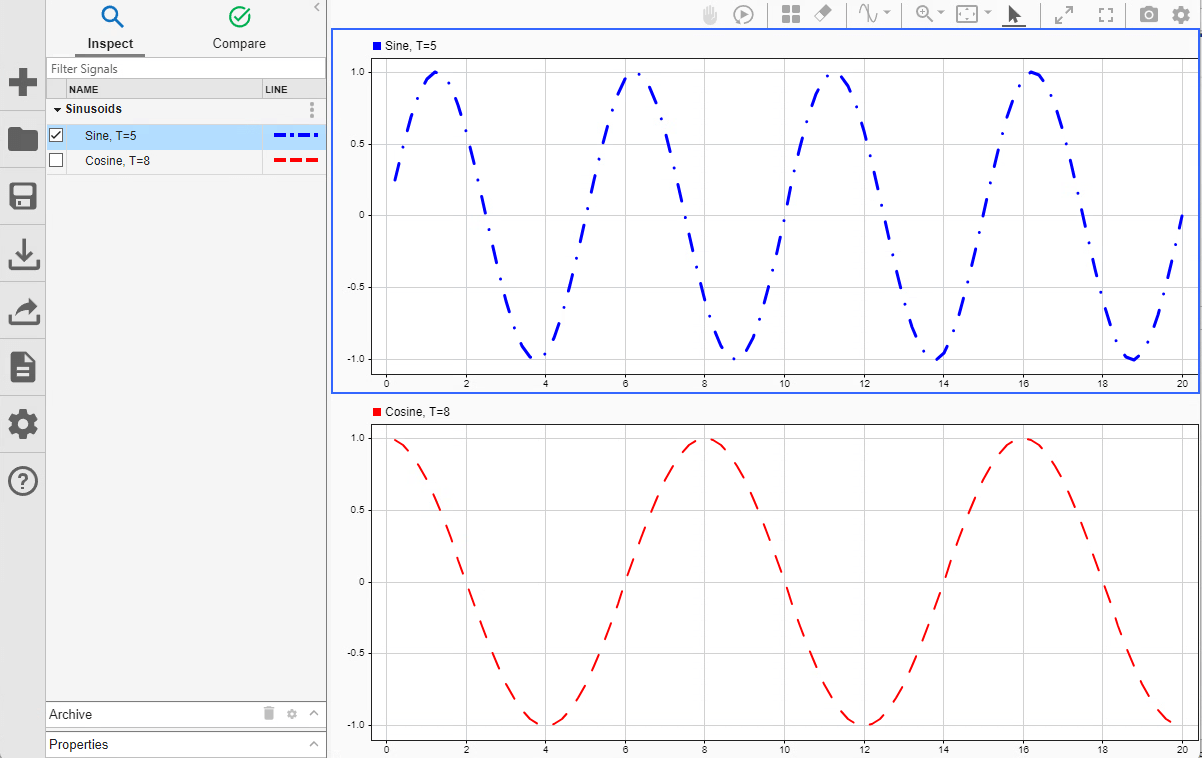# Simulation Data Inspector

Inspect and compare data and simulation results to validate and iterate model designs

## Description

The Simulation Data Inspector visualizes and compares multiple kinds of data.

Using the Simulation Data Inspector, you can inspect and compare time series data at multiple stages of your workflow. This example workflow shows how the Simulation Data Inspector supports all stages of the design cycle:

1. Run a simulation in a model configured to log data to the Simulation Data Inspector, or import data from the workspace or a file. You can view and verify model input data or inspect logged simulation data while iteratively modifying your model diagram, parameter values, or model configuration.

2. Inspect Simulation Data

Plot signals on multiple subplots, zoom in and out on specified plot axes, and use data cursors to understand and evaluate the data. You can choose from several visualizations, such as time, array, map, sparklines, and XY plots. For more information on presenting data effectively, see Create Plots Using the Simulation Data Inspector.

3. Compare individual signals or simulation runs and analyze your comparison results with relative, absolute, and time tolerances. The compare tools in the Simulation Data Inspector facilitate iterative design and allow you to highlight signals that do not meet your tolerance requirements. For more information about the comparison operation, see How the Simulation Data Inspector Compares Data.

4. Save and Share Simulation Data Inspector Data and Views

Share your findings with others by saving Simulation Data Inspector data and views.

You can also harness the capabilities of the Simulation Data Inspector from the command line. For more information, see Inspect and Compare Data Programmatically.

## Open the Simulation Data Inspector

• Simulink® Toolstrip: On the Simulation tab, under Review Results, click .

• From a model: click the streaming badge on a signal to open the Simulation Data Inspector and plot the signal.

• MATLAB® command prompt:

## Examples

expand all

Create a run, add data to it, and then view the data in the Simulation Data Inspector.

Create Data for Run

Create `timeseries` objects to contain data for a sine signal and a cosine signal. Give each `timeseries` object a descriptive name.

```time = linspace(0,20,100); sine_vals = sin(2*pi/5*time); sine_ts = timeseries(sine_vals,time); sine_ts.Name = "Sine, T=5"; cos_vals = cos(2*pi/8*time); cos_ts = timeseries(cos_vals,time); cos_ts.Name = "Cosine, T=8";```

Use the `Simulink.sdi.view` function to open the Simulation Data Inspector.

`Simulink.sdi.view`

To import data into the Simulation Data Inspector from the workspace, create a `Simulink.sdi.Run` object using the `Simulink.sdi.Run.create` function. Add information about the run to its metadata using the `Name` and `Description` properties of the `Run` object.

```sinusoidsRun = Simulink.sdi.Run.create; sinusoidsRun.Name = "Sinusoids"; sinusoidsRun.Description = "Sine and cosine signals with different frequencies";```

Use the `add` function to add the data you created in the workspace to the empty run.

`add(sinusoidsRun,"vars",sine_ts,cos_ts);`

Plot Data in Simulation Data Inspector

Use the `getSignalByIndex` function to access `Simulink.sdi.Signal` objects that contain the signal data. You can use the `Simulink.sdi.Signal` object properties to specify the line style and color for the signal and plot the signal in the Simulation Data Inspector. Specify the `LineColor` and `LineDashed` properties for each signal.

```sine_sig = getSignalByIndex(sinusoidsRun,1); sine_sig.LineColor = [0 0 1]; sine_sig.LineDashed = "-."; cos_sig = sinusoidsRun.getSignalByIndex(2); cos_sig.LineColor = [1 0 0]; cos_sig.LineDashed = "--";```

Use the `Simulink.sdi.setSubPlotLayout` function to configure a `2`-by-`1` subplot layout in the Simulation Data Inspector plotting area. Then, use the `plotOnSubplot` function to plot the sine signal on the top subplot and the cosine signal on the lower subplot.

```Simulink.sdi.setSubPlotLayout(2,1); plotOnSubPlot(sine_sig,1,1,true); plotOnSubPlot(cos_sig,2,1,true);```Close Simulation Data Inspector and Save Your Data

When you finish inspecting the plotted signal data, you can close the Simulation Data Inspector and save the session to an MLDATX file.

`Simulink.sdi.close("sinusoids.mldatx")`

You can use the `Simulink.sdi.plot` function to plot simulation results in the Simulation Data Inspector. Open the model `vdp`, which models the second-order Van der Pol differential equation. For more information about the model, see Van der Pol Oscillator.

```mdl = "vdp"; open_system(mdl)```Simulate the model. The model logs two signals: `x1` and `x2`. Simulation results are stored in a single `SimulationOutput` object named `out`.

`out = sim(mdl);`

Use the `Simulink.sdi.plot` function to open the Simulation Data Inspector and plot the results.

`Simulink.sdi.plot(out);`You can use the Simulation Data Inspector programmatic interface to modify a parameter for the same signal in multiple runs. This example adds an absolute tolerance of `0.1` to a signal in all four runs of data.

First, clear the workspace and load the Simulation Data Inspector session with the data. The session includes logged data from four simulations of a Simulink® model of a longitudinal controller for an aircraft.

```Simulink.sdi.clear Simulink.sdi.load('AircraftExample.mldatx');```

Use the `Simulink.sdi.getRunCount` function to get the number of runs in the Simulation Data Inspector. You can use this number as the index for a for loop that operates on each run.

`count = Simulink.sdi.getRunCount;`

Then, use a for loop to assign the absolute tolerance of `0.1` to the first signal in each run.

```for a = 1:count runID = Simulink.sdi.getRunIDByIndex(a); aircraftRun = Simulink.sdi.getRun(runID); sig = getSignalByIndex(aircraftRun,1); sig.AbsTol = 0.1; end```

## Programmatic Use

expand all

`Simulink.sdi.view` opens the Simulation Data Inspector from the MATLAB command line.

## Version History

Introduced in R2010b